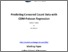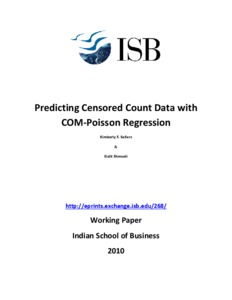# Predicting Censored Count Data with COM-Poisson Regression

Sellers, K F and Shmueli, G (2010) Predicting Censored Count Data with COM-Poisson Regression. Working Paper. Indian School of Business, Hyderabad.Preview
| Preview

## Abstract

Censored count data are encountered in many applications, often due to a data collection mechanism that introduces censoring. A common example is questionnaires with question answers of the type 0,1,2,3. We consider the problem of predicting a censored output variable Y, given a set of complete predictors X. The common solution would be to use adaptations for Poisson or negative binomial regression models that account for the censoring. We study two alternatives that allow for both over- and under-dispersion: Conway-Maxwell-Poisson (COM-Poisson) regression, and generalized Poisson regression models, each with adaptations for censoring. We compare the predictive power of these models by applying them to a German panel dataset on fertility, where we introduce censoring of di�erent levels into the outcome variable. We explore two additional variants: (1) using the mean versus the median of the predictive count distribution, and (2) ensembles of COM-Poisson models based on the parametric and non-parametric bootstrap

ISB Creators:
ISB CreatorsORCiD
Shmueli, GUNSPECIFIED
Item Type: Monograph (Working Paper)
Uncontrolled Keywords: over-dispersion, under-dispersion, predictive distribution, mean versus median predictions, ensemblesView Item